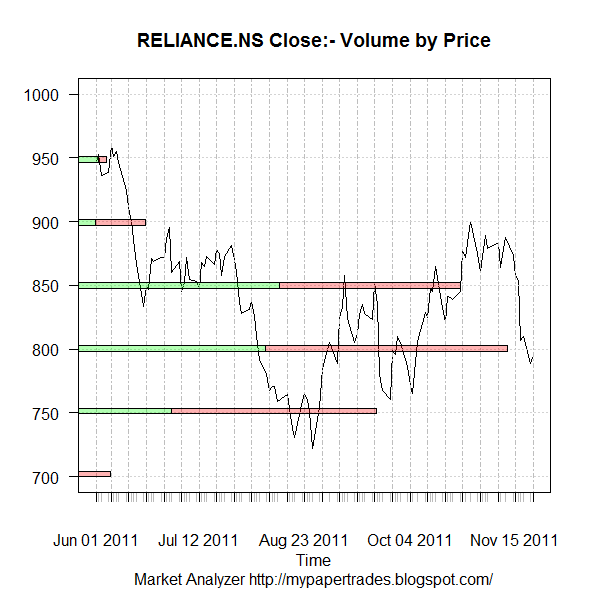Want to share your content on R-bloggers? click here if you have a blog, or here if you don't.

R-Bloggers is a wonderful site which offers some great ideas for analysis.

While I have been busy of late, hence could not do much with R, I was inspired by this post by Eric Nguyen on Volume by Price chart.

This chart can be used with a great effect in ones trading strategies. It helps in identifying the support and resistance zones, and a breakout from these levels used as entry/exit points
Extending the work done by Eric, and making few changes in his original code, here is what I have achieved.Reliance EOD Volume by Price chart
It can now be visually observed the role of volume in providing support/resistance zones.

Future Work: Make animation-like effect to show case the strength offered by volumes at various price levels over a period of time.

Not the best way to code, here is the workable version for more experimentation and extension

`library(quantmod)############################################################### #Original Code#http://blog.datapunks.com/2011/10/volume-by-price-charts-with-r-first-attempt/ #Change the ticker to get chart of any "yahoo" symbolticker = "RELIANCE.NS" symbol <- getSymbols(ticker)stock <- xts(get(symbol)) #remove stock namenames(stock)[names(stock)==paste(symbol,'.Open',sep="")] <- 'Open' names(stock)[names(stock)==paste(symbol,'.Close',sep="")] <- 'Close' names(stock)[names(stock)==paste(symbol,'.Volume',sep="")] <- 'Volume' names(stock)[names(stock)==paste(symbol,'.Adjusted',sep="")] <- 'Adjusted' names(stock)[names(stock)==paste(symbol,'.High',sep="")] <- 'High' names(stock)[names(stock)==paste(symbol,'.Low',sep="")] <- 'Low' #Add Positive and Negative Volumesstock\$posVbP <- Vo(stock[which(Lag(Cl(stock)) <= Cl(stock))])stock\$negVbP <- Vo(stock[which(Lag(Cl(stock)) > Cl(stock))])   #Since NAs got generated, replace NAs with 0.stock[is.na(stock)] <- 0 #Subset for data since May 2011myQ <- stock['2011-06::']  #Define function to add positive and negative volumes by pricespVolBlock <- function(x) sum(myQ\$posVbP[myQ\$t==x])nVolBlock <- function(x) sum(myQ\$negVbP[myQ\$t==x]) funcPriceByVol <- function(x){ myDiv <- 50         #Divisor for stock myQHi <- as.integer(ceiling(max(Cl(myQ))/myDiv)*myDiv)  #Identify High of Series myQLo <- as.integer(floor(min(Cl(myQ))/myDiv)*myDiv) #Identify Low of Series  myBreaks <- as.integer(seq(myQLo, myQHi, by=myDiv))  #Create Breaks of interval divisor  # Identify and assign price intervals myQ\$t <<- myBreaks[findInterval(myQ\$Close,myBreaks,all.inside=T)]  myVolsP <- unlist(lapply(myBreaks,pVolBlock)) #Add Positive Volumes to block myVolsN <- unlist(lapply(myBreaks,nVolBlock)) #Add Negative Volumes to block myVols <- rbind(myVolsP,myVolsN)   #Bind the Positive and Negative Volumes colnames(myVols) <- myBreaks    #Define Column Naes x=myVols} myPBV <- funcPriceByVol()  #lets Plot the graph now   plot(Cl(myQ),yaxt="n", ylab="",xlab="Time",  ylim=c(min(as.integer(colnames(myPBV))),max(as.integer(colnames(myPBV)))),  main=paste(ticker, "Close:- Volume by Price"),sub="Market Analyzer http://mypapertrades.blogspot.com/") axis(side=2,las=1) par(new=T) barplot(height=myPBV,   beside=F,horiz=T, col=c(rgb(0,1,0,alpha=.3),rgb(1,0,0,alpha=.3)),  xlim=c(0,max(myPBV[1,]+myPBV[2,])*1.1),   space=10, width=3,xaxt="n",yaxt="n",  las=2)`